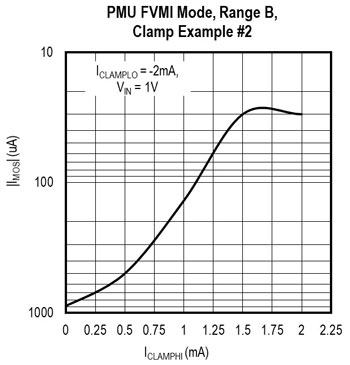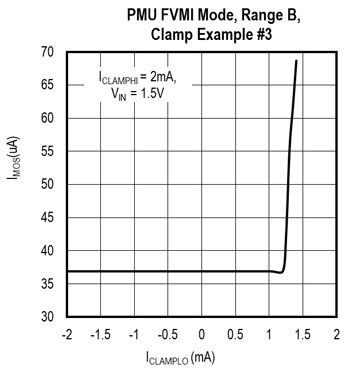# How to Set PMU Voltage and Current Clamps

### Abstract

The MAX9979 is a fully integrated pin electronics IC, consisting of DCL, PMU, and level settlers. All the blocks of the MAX9979 are enriched with features such as clamps, active load, high range of sourcing and sinking capabilities, data inversion, and differential modes. The MAX9979 parametric measurement unit (PMU) is able to both force and measure current and voltage. Setting the voltage and current clamps ensures the PMU operates in linear region. Understanding the PMU functionality and adjusting clamps according to the application is simplified with this application note.

### Introduction

Parametric measurement unit (PMU) block in the MAX9979 is responsible for forcing and measuring current and voltage. A voltage range of -1.5V to 6.5V and full-scale current range of ±2µA to ±50mA can be supported. The voltage and current clamping feature in the MAX9979 makes PMU more versatile towards its performance with some careful considerations.

It is essential to understand the PMU functionality, so this application will first cover the PMU Modes and Range of Operation. Then, the usage of PMU clamps will be covered in detail.

### PMU Modes and Range of Operation

The MAX9979 PMU can be configured in six modes of operation as shown in Table 1.

1. Force-Voltage/Measure-Voltage (FVMV)
2. Force-Voltage/Measure-Current (FVMI)
3. Force-Current/Measure-Current (FIMI)
4. Force-Current/Measure-Voltage (FIMV)
5. Force-Nothing/Measure-Voltage (FNMV)
6. Force-Nothing/Measure-Nothing (FNMN)
 Digital Input Serial Interface Bits PMU Mode LLEAKP HIZFORCE FMODE MMODE 1 1 X X FyMy* 1 1 X X FyMy* 1 0 0 X FVMy* (calibration) 1 0 0 X FVMy* (calibration) 1 0 1 0 FNMN 1 0 1 1 FNMV (calibration) 1 0 1 1 FNMV (calibration) 0 X X 0 FNMN 0 X X 1 FNMV (calibration) 0 X X 1 FNMV (calibration) *y = V or I

Based on full-scale current range selection, PMU force-current and measure-current can be operated or controlled in different current ranges through three bits of control word (RS2, RS1, and RS0) as captured in Table 2.

 Digital Input Serial Interface Bits PMU Range (IRANGE, RRANGE) LLEAKP HIZFORCE RS2 RS1 RS0 X X 0 0 0 E(±2µA, 500kO) X X 0 0 1 D(±20µA, 50kO) X X 0 1 0 C(±200µA, 5kO) X X 0 1 1 B(±2mA, 500O) X 0 1 X X B* 0 1 1 X X B* 1 1 1 X X A(±50mA, 20O) * Range A operation is not allowed for PMU high-impedance modes — PMU defaults to range B.

For more details on PMU operation, refer to PMU MODE OPERATION FOR THE MAX9979 PIN-ELECTRONICS IC.

### PMU Voltage/Current Clamps

PMU voltage or current clamps ensure that the DUT_ voltage or current cannot exceed the clamped voltage or current, respectively. PMU voltage clamps are available in FI mode of operation whereas current clamps are available in FV mode. When a PMU voltage/current clamp is active, within its limits, the MV and MI functions are still valid.

Setting CLAMPHI_ and CLAMPLO_ digital-to-analog converters (DAC) sets the high clamp voltage (VCLAMPHI) and low clamp voltage (VCLAMPLO) respectively. In FI mode, the user can set the voltage clamps directly by utilizing CLAMPHI_ and CLAMPLO_ DACs. For current clamps IIOS DAC (VIIOS) needs to be set along with CLAMPHI_ and CLAMPLO_ DACs. Clamp high current (ICLAMPHI) and clamp low current (ICLAMPLO) equations are given below.

ICLAMPHI = (VCLAMPHI - VIIOS)/RRANGE

VCLAMPHI = (ICLAMPHI × RRANGE) + VIIOS

#### Example 1:

Consider PMU in FVMI mode and range B operation.

Considering VIIOS DAC voltage is 2.5V, 1K? is the DUT load. Fix the ICLAMPHI as 2mA and ICLAMPLO as -2mA. Start VIN_ from 2V and gradually decrease. Equations 1 and 2 are utilized for the calculations in Table 3 below.

 VIN (V) VIIOS (V) ICLAMPHI (mA) ICLAMPLO (mA) CLAMPHI DAC Voltage (V) CLAMPLO DAC Voltage(V) VDUT (V) IDUT (mA) IMOS (uA) 2 2.5 +2 -2 3.5 1.5 1.86598 1.92857 71.43 1.5 2.5 +2 -2 3.5 1.5 1.4969 1.5329 -32.9 1 2.5 +2 -2 3.5 1.5 0.9996 1.0299 -29.9 0.5 2.5 +2 -2 3.5 1.5 0.4992 0.5012 -1.2 0 2.5 +2 -2 3.5 1.5 0.0082 0.0085 -8.5

As VIN_ decreases gradually, VDUT_ and IDUT also decrease. The MAX9979 PMU has measure current offset (IMOS) of ±1 % FSR (as mentioned in the EC table of the data sheet). Since PMU is in range B operation (FSR = 4mA) the maximum current offset expected is ±40µA. If the IDUT has offset more than ±40µA then the output is not desirable. In table 3, the teal color readings are desirable, and the orange color readings are not desirable. For the PMU, there are two conditions that need to be met.To maintain the linearity in FI mode:

(VCLAMPLO + 0.5V) = VDUT = (VCLAMPHI – 0.5V)

To maintain the linearity in FV mode:

(ICLAMPLO + 10% FSR) = IDUT = (ICLAMPHI – 10%FSR)

#### Example 2:

Consider PMU in FVMI mode and range B operation.

FMODE = 0, MMODE = 0, RRANGE = 500?, FSR = 4mA

Considering VIIOS DAC voltage is 2.5V, 1K? is the DUT_ load. Fix the VIN_ DAC voltage as 1V. Change the clamp current from 2mA to 0mA. Equations 1 and 2 are used for the calculations in Table 4 below.

Expected IDUT = VDUT/RLOAD = 1V/1K? = ±1mA

Note: ±sign indicates that the MAX9979 device can either source or sink the current.

In range B operation: 10% FSR = 10% of 4mA = 0.4mA

From equation 4, assume the following about the device:

IDUT = (ICLAMPHI – 10% FSR)

IDUT + 10% FSR = ICLAMPHI

ICLAMPHI = 1mA + 0.4mA = 1.4mA

Similarly, ICLAMPLO = -1.4mA

 VIN (V) VIIOS (V) ICLAMPHI (mA) ICLAMPLO (mA) CLAMPHI DAC Voltage (V) CLAMPLO DAC Voltage(V) VDUT (V) IDUT (mA) IMOS (uA) 1 2.5 2 -2 3.5 1.5 0.9996 1.0299 29.9 1 2.5 1.5 -2 3.25 1.5 0.9996 1.0299 29.9 1 2.5 1 -2 3 1.5 0.8642 0.8623 -137.7 1 2.5 0.5 -2 2.75 1.5 0.4911 0.5018 -498.2 1 2.5 0 -2 2.5 1.5 0.1117 0.1155 -884.5

VIN is constant at 1V. In FVMI mode, we expect that VDUT = 1V. Therefore IDUT = VDUT/RDUT = 1V/1K? = +1mA. Observe in the orange color rows, that when ICLAMPHI is less than 1.4mA, the VDUT and IDUT readings are not the expected values. In this case, the IDUT offset error is more than ±40uA.#### Example 3:

Consider PMU in FVMI mode and range B operation.

FMODE = 0, MMODE = 0, RRANGE = 500?, FSR = 4mA

Considering VIIOS DAC voltage is 2.5V, 1K? is the DUT_ load. Fix the VIN_ DAC voltage as 1.5V and ICLAMPHI as 2mA. Change the ICLAMPLO from -2mA to 1.4mA. Equations 1 and 2 are used for the calculations in Table 5 below.

 VIN (V) VIIOS (V) ICLAMPHI (mA) ICLAMPLO (mA) CLAMPHI DAC Voltage (V) CLAMPLO DAC Voltage(V) VDUT (V) IDUT (mA) IMOS (uA) 1.5 2.5 2 -2 3.5 1.5 1.4912 1.5369 36.9 1.5 2.5 2 -1 3.5 2 1.4912 1.5369 36.9 1.5 2.5 2 0 3.5 2.5 1.4912 1.5369 36.9 1.5 2.5 2 1 3.5 3 1.4912 1.5369 36.9 1.5 2.5 2 1.2 3.5 3.1 1.4912 1.5369 36.9 1.5 2.5 2 1.4 3.5 3.2 1.5174 1.5687 68.7

From equation 4,

(ICLAMPLO + 10% FSR) = IDUT = (ICLAMPHI – 10% FSR)

(ICLAMPLO + 10% FSR) = (ICLAMPHI – 10% FSR)

ICLAMPHI – ICLAMPLO = 20% FSR

VCLAMPHI – VCLAMPLO = (20% FSR) × RRANGE; from equations 1 and 2.#### Example 4:

Consider PMU in FIMV mode and range B operation.

FMODE = 1, MMODE = 1, RRANGE = 500?, FSR = 4mA

Considering VIIOS DAC voltage is 2.5V, 1K? is the DUT_ load. Fix the VIN DAC voltage as 5.5V. Change the clamp voltages from ±2.5V to ±1.5V.

When PMU is in FI mode, the voltage clamp comes into the picture. From equation 3,

(VCLAMPLO + 0.5V) = VDUT = (VCLAMPHI – 0.5V).

In FI mode, the current flowing out of DUT is calculated per equation 5 below.

DUT = (VIN – VIIOS)/(4 × RRANGE)

Where;

IDUT = (5.5 – 2.5)/(4 × 500)

IDUT = 1.5mA

VDUT = IDUT × RLOAD = 1.5mA × 1K? = 1.5V

 VIN (V) VIIOS (V) VCLAMPHI (V) VCLAMPLO (V) VDUT (V) IDUT (mA) VMOS (uA) 5.5 2.5 2.5 -2.5 1.487 1.498 -13 5.5 2.5 2 -2 1.487 1.498 -13 5.5 2.5 1.5 -1.5 1.393 1.441 -107

The expected DUT voltage is 1.5V and the clamp voltage should be 0.5V higher than the expected DUT voltage. When the clamp voltage is the same as the DUT voltage, the DUT voltage starts decreasing (see the orange rows in the chart). The measure of voltage offset (VMOS) increases more than 25mV.

### Summary

This application note provides the user with guidelines on operating PMU in linear region. It is quite simple, with the below two equations:

To maintain linearity in FI mode:

(VCLAMPLO + 0.5V) = VDUT = (VCLAMPHI – 0.5V)

To maintain linearity in FV mode:

(ICLAMPLO + 10% FSR) = IDUT = (ICLAMPHI – 10% FSR)

### Appendix – Abbreviations:

FI – Force current

FV – Force voltage

ICLAMPHI – High clamp current

ICLAMPLO – Low clamp current

IDUT – DUT output current

IRANGE – Current range

MI – Measure current

MV – Measure voltage

PMU – Parametric measurement unit

VCLAMPHI – High clamp voltage

VCLAMPLO – Low clamp voltage

VDUT – DUT output voltage